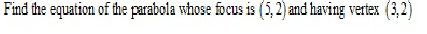# RD SHARMA Solutions for Class 11-science Maths Chapter 25 - Parabola

Page / Exercise

## Chapter 25 - Parabola Exercise Ex. 25.1

Question 1(i)Solution 1(i)Question 1(iii)Solution 1(iii)Question 1(ii)Solution 1(ii)Question 1(iv)Solution 1(iv)Question 2Solution 2Question 3(i)Solution 3(i)Question 3(ii)Solution 3(ii)Question 3(iii)Solution 3(iii)Question 3(iv)Solution 3(iv)Question 3(v)Solution 3(v)Question 4(i)Solution 4(i)Question 4(ii)Solution 4(ii)Question 4(iii)Solution 4(iii)Question 4(iv)Solution 4(iv)Question 4(v)Solution 4(v)Question 4(vi)Solution 4(vi)Question 4(vii)Solution 4(vii)Question 4(viii)Solution 4(viii)Question 4(ix)Solution 4(ix)Question 5Solution 5Question 6

Find the area of the triangle formed by the lines joining the vertex of the parabolato the ends of its latus- rectum

Solution 6Question 7Solution 7Question 8Solution 8Question 9Solution 9Question 10Solution 10Question 11Solution 11Question 12Solution 12Question 13

Find the equation of the lines joining the vertex of the parabola y2 = 6x to the point on it which have abscissa 24

Solution 13Question 14

Find the coordinates of points on the parabola y2 = 8x whose focal distance is 4

Solution 14

In given parabola

a=2

Given focal distance=a+x=4, so x=2

So points are (2, 4) and (2, -4)

Question 15

Find the length  of the lines segment joining the vertex of the parabola y2 = 4ax and a point on the parabola where the line-segment makes an angleto the axis

Solution 15Question 16

If the points (0, 4) and (0, 2) are respectively the vertex and focus of a parabola, then find the equation of the parabola.

Solution 16Question 17

If the line y = mx + 1 is tangent to the parabola y2 = 4x, then find the value of m.

Solution 17## Chapter 25 - Parabola Exercise Ex. 25VSAQ

Question 1Solution 1Question 2Solution 2Question 3Solution 3Question 4Solution 4Question 5Solution 5Question 6Solution 6Question 7Solution 7Question 8Solution 8Question 9Solution 9### STUDY RESOURCES

REGISTERED OFFICE : First Floor, Empire Complex, 414 Senapati Bapat Marg, Lower Parel, Mumbai - 400013, Maharashtra India.